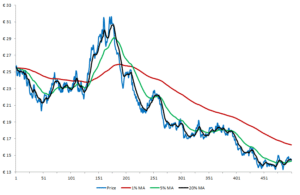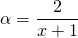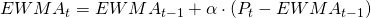# Exponentially weighted moving average

The exponentially weighted moving average, or in short the exponential moving average, is a moving average technical indicator which uses an exponential weighting scheme of past prices. Compared to the simple moving average indicator, this metric puts more weight on recent prices.

## Dual exponentially weighted moving average

Similar like the dual moving average trading system based on the simple moving average, the dual exponentially weighted moving average uses 2 indicators to generate buy and sell signals. The first moving average uses a smaller lag parameter and imposes an exponential weighting scheme. Therefore, this indicator follows closely the price series but also evolves more erratic over time. The second indicator uses a larger lag parameter and thus evolves more smoothly over time but follows less recent price changes. Technical analysts argue that the crossovers generates buy signals whenever the short moving average crosses the long one from below upwards. Sell signals are generated when the short moving average crosses the longer one from above downwards.## Exponentially weighted moving average formula

How to calculate an exponentially weighted moving average? This is somewhat more tricky than the calculation of a simple moving average as a result of a more complicated weighting scheme. First a memory parameter, α, need to be determined.  This parameter needs to have a value between 0 and 1. The larger this parameter is, the longer its memory and the more time it will take to incorporate new changes. This parameter can be directly imposed, or calculated based on a required amount of look back days as shown in the formula below.## Summary

The EWMA is a specific type of moving average that puts more weight on recent price. As such, this indicator will follow more closely the price series than a simple moving average.